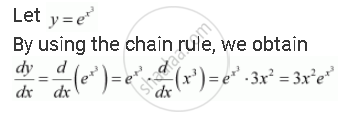Share

# Differentiate the Following W.R.T. X: E^(X^3) - CBSE (Commerce) Class 12 - Mathematics

ConceptExponential and Logarithmic Functions

#### Question

Differentiate the following w.r.t. x: e^(x^3)

#### SolutionIs there an error in this question or solution?

#### APPEARS IN

NCERT Solution for Mathematics Textbook for Class 12 (2018 to Current)
Chapter 5: Continuity and Differentiability
Q: 3 | Page no. 174

#### Video TutorialsVIEW ALL 

Solution Differentiate the Following W.R.T. X: E^(X^3) Concept: Exponential and Logarithmic Functions.
S